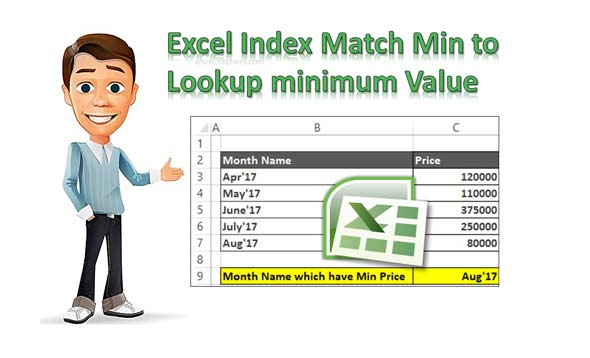# Excel Index Match Min to Lookup minimum Value

Finding the smallest value in an important thing. Excel Index Match Min function allows you to lookup the minimum value in active worksheet.

Finding the lowest value is an important task in Excel worksheet. You can use Excel Index Match Min function to lookup minimum value. If you have large worksheet data in which you want to find the minimum value which match the condition as per your need. There are lot of ways from which you can find out lowest value which match the given condition. You can use Index and Match function along with Min to find out lowest value in the active worksheet.

### Index Function in Excel

Index function return a value or the reference number to a value from given range of cells or table array.

INDEX(array, row_num, [column_num])

Array : It is a range of cells or an array constant.

Row_num : Selects the row number in an array from which to return a value. If Row_num is omitted, Column_num is required.

Column_num : It is an optional. Selects the column number in an array from which to return a value. If Column_num is omitted, Row_num is required.

### Match Function in Excel

This function is used to returns the relative position of an item in the given array or range of cells that match a specific value in specific order.

MATCH (lookup value, lookup array, [match_type] )

lookup_value: The value that you want to match in lookup_array. The lookup_value argument can be any number, text, logical value or a cell reference to a number, text, or logical value.

lookup_array: It is range of cells being searched.

match_type: It is an optional. The number -1, 0, or 1. The match_type argument specifies how Excel matches lookup_value with values in lookup_array. By default value for this argument is 1.

### Min Function in Excel

The Excel MIN function allows you to return the smallest value from set of numeric values.

MIN( number1, [number2], … )

Number1, number2, … Number1 is an optional argument. 1 to 255 numbers for which you want to find the minimum value.

## Steps to use Excel Index Match Min to Lookup Minimum ValueStep 1: Create the following table to use the Excel Index, Match and Min function to get the smallest value which match the condition.

Step 2: Type the following given function in cell C9 =INDEX(B3:B7,MATCH(MIN(C3:C7),C3:C7,0)) and press enter. Now you can find the month name which has lowest price in active worksheet.

I hope after reading this guide you can easily use Excel Index Match Min to Lookup minimum value. If you have any suggestion regarding this guide then please write us in the comment box. Thanks to all.